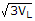# Electrical Engineering - Three-Phase Systems in Power Applications

### Exercise :: Three-Phase Systems in Power Applications - General Questions

11.

A three-phase generator is connected to three 90load resistors. Each coil generates 120 V ac. A common neutral line exists. How much current flows through the common neutral line?

 A. 0 A B. 1.33 A C. 3.99 A D. 2.66 A

Explanation:

No answer description available for this question. Let us discuss.

12.

Compare the total copper cross sections in terms of current-carrying capacity for a single-phase and a three-phase 120 V system with effective load resistance of 15.

 A. single-phase 32 A; three-phase 16 A B. single-phase 16 A; three-phase 8 A C. single-phase 8 A; three-phase 4 A D. single-phase 16 A; three-phase 0 A

Explanation:

No answer description available for this question. Let us discuss.

13.

If in a Y-connected ac generator, each phase voltage has a magnitude of 90 VRMS, what is the magnitude of each line voltage?

 A. 0 V B. 90 V C. 156 V D. 180 V

Explanation:

No answer description available for this question. Let us discuss.

14.

Polyphase generators produce simultaneous multiple sinusoidal voltages that are separated by

 A. certain constant phase angles B. certain constant frequencies C. certain constant voltages D. certain constant currents

Explanation:

No answer description available for this question. Let us discuss.

15.

In a balanced three-phase load, each phase has

 A. an equal amount of power B. one-third of total power C. two-thirds of total power D. a power consumption equal toIL